# 利用 CatBoost 算法寻找外汇市场的季节性模式

11 三月 2021, 08:41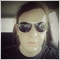0
845

### 时间过滤器函数

```def time_filter(data, count):
# filter by hour
hours=
if data.index[count].hour not in hours:
return False

# filter by day of week
days = 
if data.index[count].dayofweek not in days:
return False

return True
```

add_labels 函数现在接收这个条件作为输入。在Python中，函数是一级对象，因此可以将它们作为参数安全地传递给其他函数。

```def add_labels(dataset, min, max, filter=time_filter):
labels = []
for i in range(dataset.shape-max):
rand = random.randint(min, max)
curr_pr = dataset['close'][i]
future_pr = dataset['close'][i + rand]
if filter(dataset, i):
if future_pr + MARKUP < curr_pr:
labels.append(1.0)
elif future_pr - MARKUP > curr_pr:
labels.append(0.0)
else:
labels.append(2.0)
else:
labels.append(2.0)
dataset = dataset.iloc[:len(labels)].copy()
dataset['labels'] = labels
dataset = dataset.dropna()
dataset = dataset.drop(
dataset[dataset.labels == 2].index)

return dataset
```

```def tester(dataset, markup=0.0, plot=False, filter=time_filter):
last_deal = int(2)
last_price = 0.0
report = [0.0]
for i in range(dataset.shape):
pred = dataset['labels'][i]
ind = dataset.index[i].hour
if last_deal == 2 and filter(dataset, i):
last_price = dataset['close'][i]
last_deal = 0 if pred <= 0.5 else 1
continue
if last_deal == 0 and pred > 0.5:
last_deal = 2
report.append(report[-1] - markup +
(dataset['close'][i] - last_price))
continue
if last_deal == 1 and pred < 0.5:
last_deal = 2
report.append(report[-1] - markup +
(last_price - dataset['close'][i]))

y = np.array(report).reshape(-1, 1)
X = np.arange(len(report)).reshape(-1, 1)
lr = LinearRegression()
lr.fit(X, y)

l = lr.coef_
if l >= 0:
l = 1
else:
l = -1

if(plot):
plt.plot(report)
plt.plot(lr.predict(X))
plt.title("Strategy performance")
plt.xlabel("the number of trades")
plt.ylabel("cumulative profit in pips")
plt.show()

return lr.score(X, y) * l
```

### 每个交易小时的探索性分析

```def exploratory_analysis():
h = [x for x in range(24)]
result = pd.DataFrame()
for _h in h:
global hours
hours = [_h]
pr = get_prices(START_DATE, STOP_DATE)
pr = add_labels(pr, min=15, max=15, filter=time_filter)
gmm = mixture.GaussianMixture(
n_components=n_compnents, covariance_type='full', n_init=1).fit(pr[pr.columns[1:]])

# iterative learning
res = []
iterations = 10
for i in range(iterations):
res.append(brute_force(10000, gmm))
print('Iteration: ', i, 'R^2: ', res[-1], ' hour= ', _h)

r = pd.DataFrame(np.array(res)[:, 0], np.full(iterations,_h))
result = result.append(r)

plt.scatter(result.index, result, c = result.index)
plt.show()
return result
```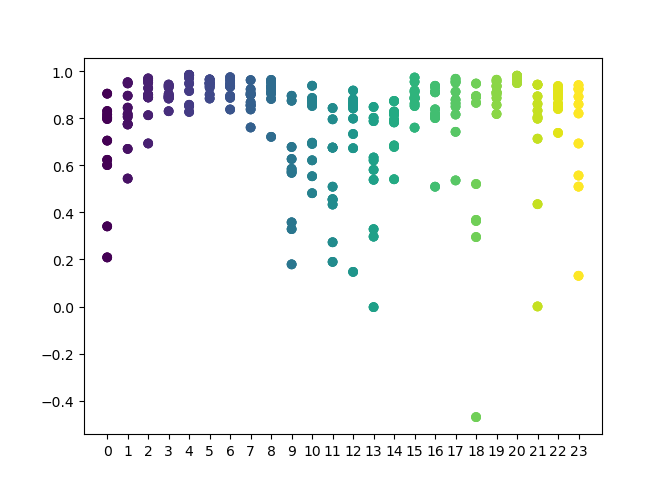X轴以小时的序号为特征。Y轴表示每次迭代的R^2分数（使用10次迭代，这意味着每小时进行一次模型再培训）。如您所见，4小时、5小时和6小时的通行证位于更近的位置，这使您对找到的模式的质量更有信心。选择原则很简单 - 点的位置和密度越高，模型就越好。例如，在9-15点的时间间隔内，图中显示了大量的点分散，模型的平均质量下降到0.6。您可以进一步选择所需的小时数，重新训练模型并在自定义测试器中查看其结果。

### 测试选择的模型

```SYMBOL = 'GBPUSD'
MARKUP = 0.00010
TIMEFRAME = mt5.TIMEFRAME_H1
START_DATE = datetime(2017, 1, 1)
TSTART_DATE = datetime(2015, 1, 1)
FULL_DATE = datetime(2015, 1, 1)
STOP_DATE = datetime(2021, 1, 1)
```

```hours = [3,4,5,6]
# make dataset
pr = get_prices(START_DATE, STOP_DATE)
pr = add_labels(pr, min=15, max=15, filter=time_filter)
tester(pr, MARKUP, plot=True, filter=time_filter)

# perform GMM clasterizatin over dataset
# gmm = mixture.BayesianGaussianMixture(n_components=n_compnents, covariance_type='full').fit(X)
gmm = mixture.GaussianMixture(
n_components=n_compnents, covariance_type='full', n_init=1).fit(pr[pr.columns[1:]])

# iterative learning
res = []

for i in range(10):
res.append(brute_force(10000, gmm))
print('Iteration: ', i, 'R^2: ', res[-1])

# test best model
res.sort()
test_model(res[-1])
```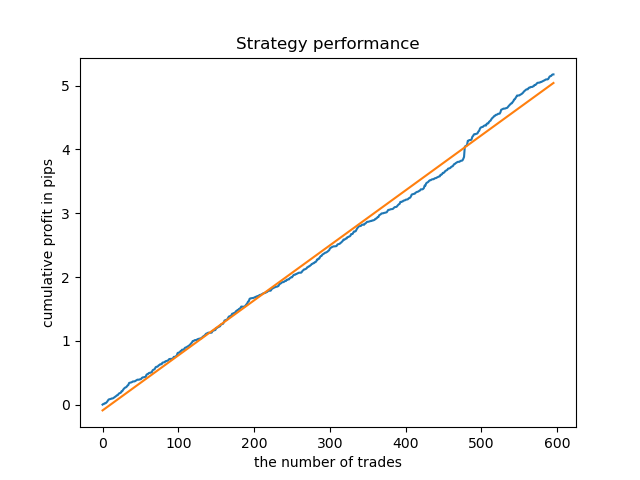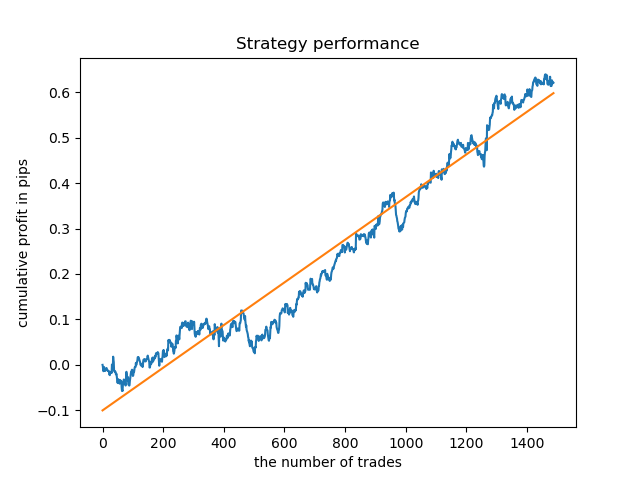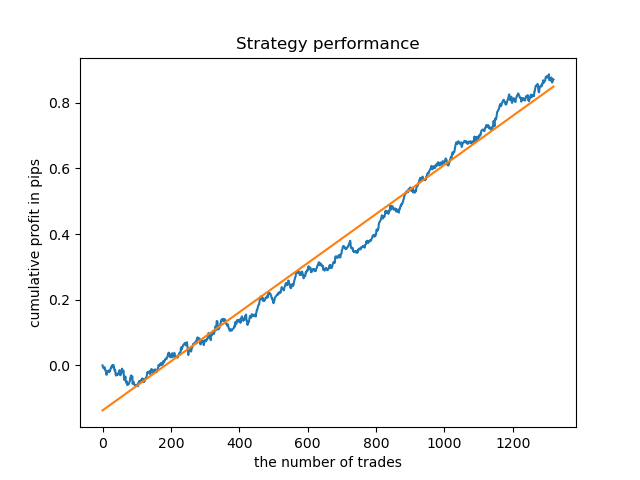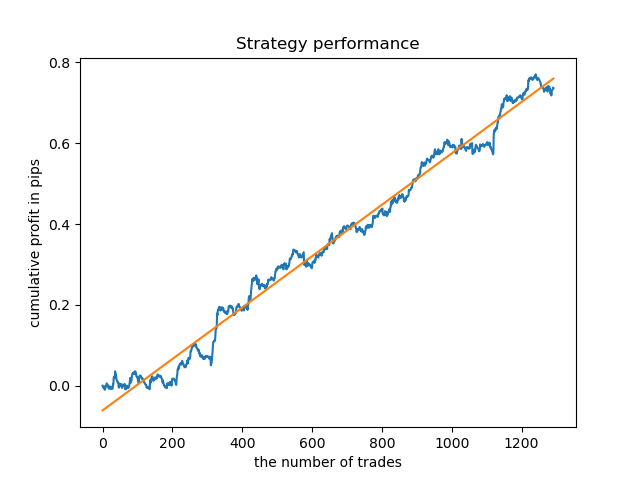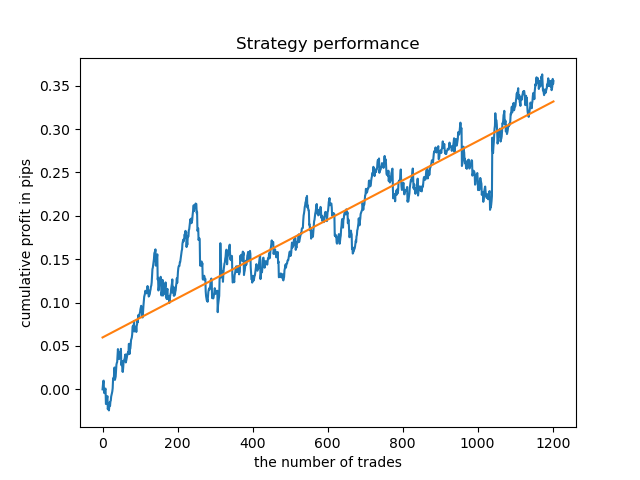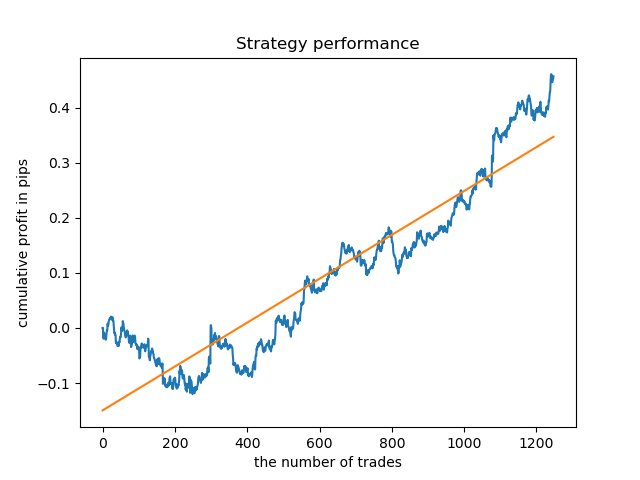### 每个交易日的探索性分析

```def time_filter(data, count):
# filter by day of week
global hours
if data.index[count].dayofweek not in hours:
return False
return True
```

```def exploratory_analysis():
h = [x for x in range(5)]
```

`pr = add_labels(pr, min=15, max=15, filter=time_filter)`

```Iteration:  0 R^2:  0.5297625368835237  hour=  0
Iteration:  1 R^2:  0.8166096906047893  hour=  0
Iteration:  2 R^2:  0.9357674260125702  hour=  0
Iteration:  3 R^2:  0.8913802241811986  hour=  0
Iteration:  4 R^2:  0.8079720208707672  hour=  0
Iteration:  5 R^2:  0.8505663844866759  hour=  0
Iteration:  6 R^2:  0.2736870273207084  hour=  0
Iteration:  7 R^2:  0.9282442121644887  hour=  0
Iteration:  8 R^2:  0.8769775718602929  hour=  0
Iteration:  9 R^2:  0.7046666925774866  hour=  0
Iteration:  0 R^2:  0.7492883761480897  hour=  1
Iteration:  1 R^2:  0.6101962958733655  hour=  1
Iteration:  2 R^2:  0.6877652983219245  hour=  1
Iteration:  3 R^2:  0.8579669286548137  hour=  1
Iteration:  4 R^2:  0.3822441930760343  hour=  1
Iteration:  5 R^2:  0.5207801806491617  hour=  1
Iteration:  6 R^2:  0.6893157850263495  hour=  1
Iteration:  7 R^2:  0.5799059801202937  hour=  1
Iteration:  8 R^2:  0.8228326786957887  hour=  1
Iteration:  9 R^2:  0.8742262956151615  hour=  1
Iteration:  0 R^2:  0.9257707800422799  hour=  2
Iteration:  1 R^2:  0.9413981795880517  hour=  2
Iteration:  2 R^2:  0.9354221623113591  hour=  2
Iteration:  3 R^2:  0.8370429185837882  hour=  2
Iteration:  4 R^2:  0.9142875737195697  hour=  2
Iteration:  5 R^2:  0.9586871067966855  hour=  2
Iteration:  6 R^2:  0.8209392060391961  hour=  2
Iteration:  7 R^2:  0.9457287035542066  hour=  2
Iteration:  8 R^2:  0.9587372191281025  hour=  2
Iteration:  9 R^2:  0.9269140213952402  hour=  2
Iteration:  0 R^2:  0.9001009579436263  hour=  3
Iteration:  1 R^2:  0.8735623527502183  hour=  3
Iteration:  2 R^2:  0.9460714774572146  hour=  3
Iteration:  3 R^2:  0.7221720163838841  hour=  3
Iteration:  4 R^2:  0.9063579778744433  hour=  3
Iteration:  5 R^2:  0.9695391076372475  hour=  3
Iteration:  6 R^2:  0.9297881558889788  hour=  3
Iteration:  7 R^2:  0.9271590681844957  hour=  3
Iteration:  8 R^2:  0.8817985496711311  hour=  3
Iteration:  9 R^2:  0.915205007218742   hour=  3
Iteration:  0 R^2:  0.9378516360378022  hour=  4
Iteration:  1 R^2:  0.9210968481902528  hour=  4
Iteration:  2 R^2:  0.9072205941748894  hour=  4
Iteration:  3 R^2:  0.9408826184927528  hour=  4
Iteration:  4 R^2:  0.9671981453714584  hour=  4
Iteration:  5 R^2:  0.9625144032389237  hour=  4
Iteration:  6 R^2:  0.9759244293257822  hour=  4
Iteration:  7 R^2:  0.9461473783201281  hour=  4
Iteration:  8 R^2:  0.9190627222826241  hour=  4
Iteration:  9 R^2:  0.9130350931314233  hour=  4
```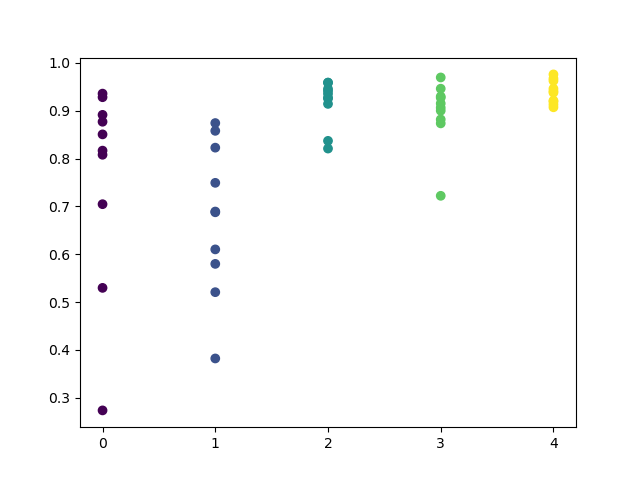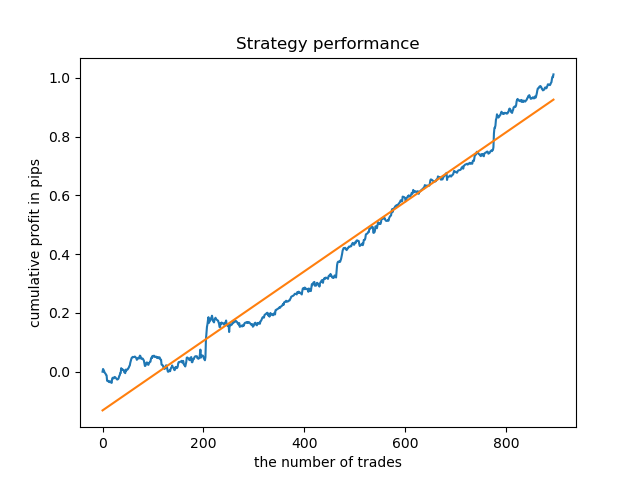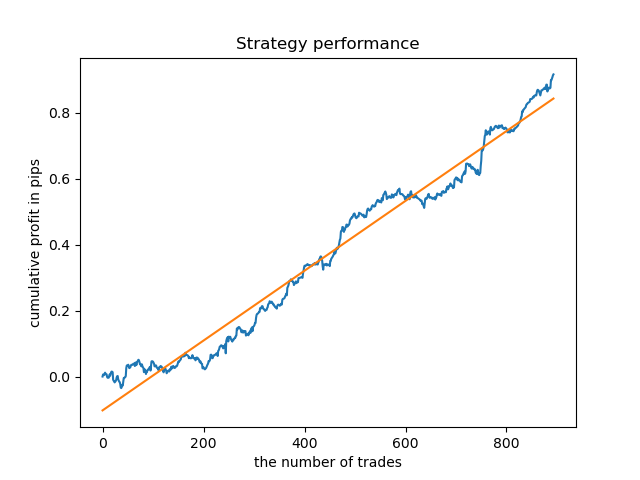```pr = add_labels(pr, min=5, max=25, filter=time_filter)
gmm = mixture.GaussianMixture(
n_components=n_compnents, covariance_type='full', n_init=1).fit(pr[pr.columns[1:]])

# iterative learning
res = []
iterations = 20
```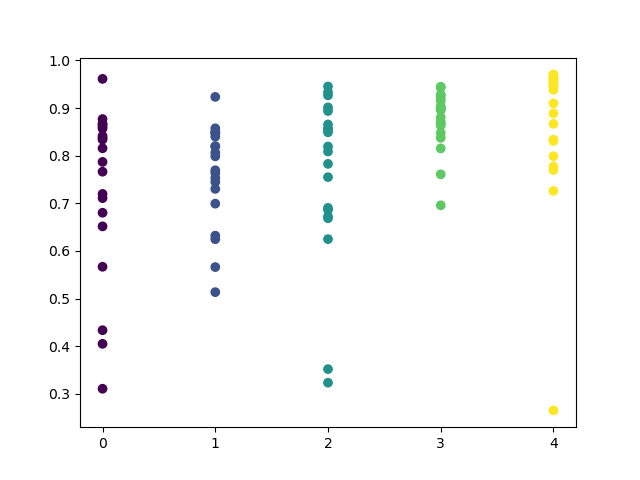```hours = 
# make dataset
pr = get_prices(START_DATE, STOP_DATE)
pr = add_labels(pr, min=5, max=25, filter=time_filter)
tester(pr, MARKUP, plot=True, filter=time_filter)

# perform GMM clasterizatin over dataset
# gmm = mixture.BayesianGaussianMixture(n_components=n_compnents, covariance_type='full').fit(X)
gmm = mixture.GaussianMixture(
n_components=n_compnents, covariance_type='full', n_init=1).fit(pr[pr.columns[1:]])

# iterative learning
res = []
for i in range(10):
res.append(brute_force(10000, gmm))
print('Iteration: ', i, 'R^2: ', res[-1])

# test best model
res.sort()
test_model(res[-1])
```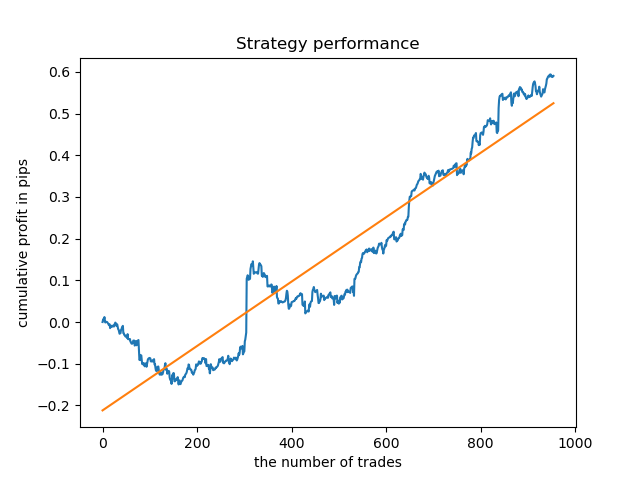### 交易期间对模型质量影响的评估

```def deals_frequency_analyzer():
freq = [x for x in range(1, 50)]
result = pd.DataFrame()
for _h in freq:
pr = get_prices(START_DATE, STOP_DATE)
pr = add_labels(pr, min=_h, max=_h, filter=time_filter)
gmm = mixture.GaussianMixture(
n_components=n_compnents, covariance_type='full', n_init=1).fit(pr[pr.columns[1:]])

# iterative learning
res = []
iterations = 5
for i in range(iterations):
res.append(brute_force(10000, gmm))
print('Iteration: ', i, 'R^2: ', res[-1], ' deal lifetime = ', _h)

r = pd.DataFrame(np.array(res)[:, 0], np.full(iterations,_h))
result = result.append(r)

plt.scatter(result.index, result, c = result.index)
plt.xticks(np.arange(0, len(freq)+1, 1))
plt.title("Performance by deals lifetime")
plt.xlabel("deals frequency")
plt.ylabel("R^2 estimation")
plt.show()
return result
```

“freq”列表包含要迭代的交易时期的值。我在 GBPUSD 对的第5个小时执行了这个迭代，这是结果。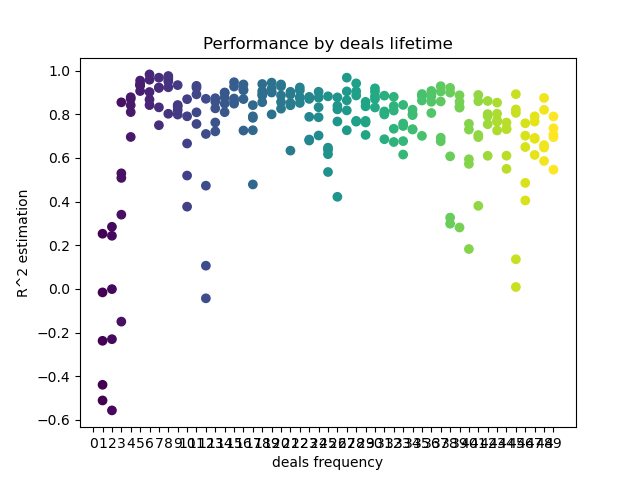X轴显示交易频率，或者更确切地说，以柱数为单位显示其时间。Y轴表示每个过程的R^2分数。如您所见，0-5 个柱的太短交易对模型性能有负面影响，而15到23个柱的时间是最佳的。更长的交易（超过30个柱）会使结果变坏。有一个小集群，其交易时间为6-9个柱，得分最高。让我们尝试用这些生存期值来训练模型，并将结果与其他集群进行比较。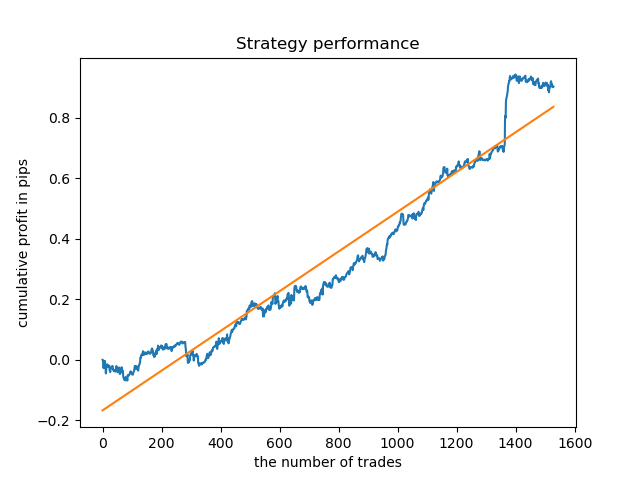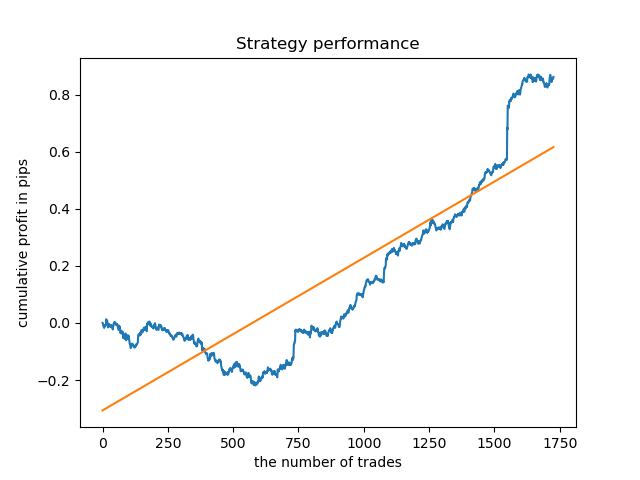```pr = add_labels(pr, min=15, max=23, filter=time_filter)

```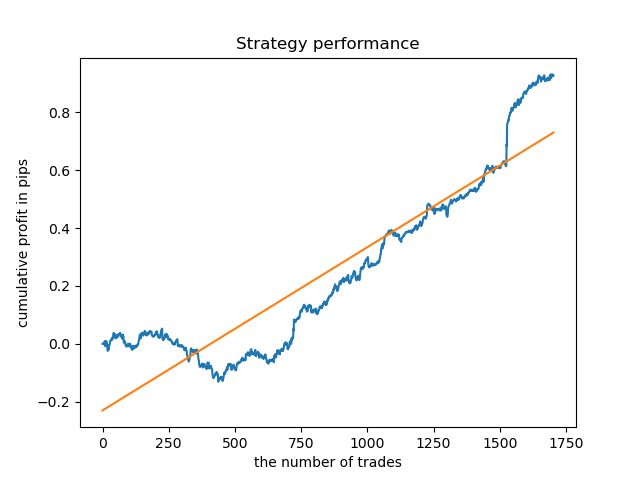### 在较长的历史上进行测试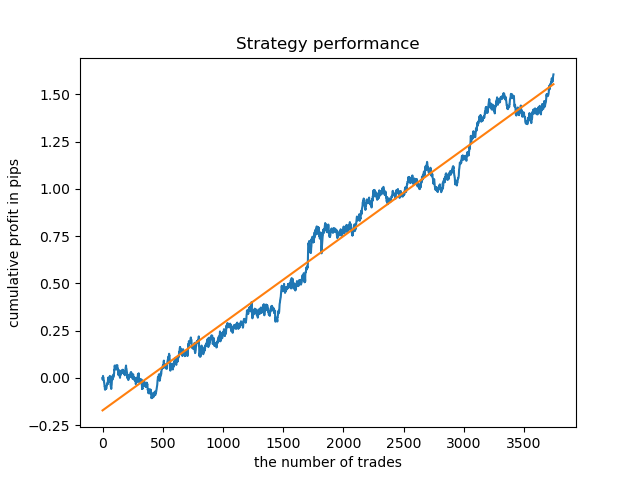### 结论

#### 该作者的其他文章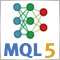神经网络变得轻松（第九部分）：操作归档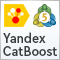直推和主动机器学习中的梯度提升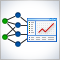神经网络在交易中的实际应用 (第二部分). 计算机视觉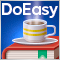DoEasy 函数库中的时间序列（第五十七·部分）：存储一次即时报价数据的对象